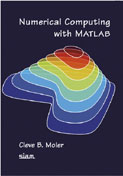# Numerical Computing with MATLAB

## by Cleve Moler.Introduction:
This is an excellent textbook on basic numerical methods, the MATLAB programming environment, and technical computing. The problems are enjoyable and interesting. I would recommend it highly to anyone who wanted . Note: the entire book, chapter by chapter can be found online here

Here are a few problems from the book I have had time to write the solutions to.

Matlab Code For Various Problems:
Chapter 7 (Ordinary Differential Equations):
• prob_7_2.m (a comparison of methods for computing compound interest numerically)
• prob_7_4.m (evaluating the error function using its differential equation)
• prob_7_4_fn.m (the error functions differential equation)
• prob_7_5.m (error experiments with RK4)
• prob_7_5_fn.m (the differential equation for a simple harmonic oscillator)
• myrk4.m (a fixed stepsize Runge-Kutta integrator (of global order 4))
• prob_7_6.m (experiments with stiff and non-stiff ODE solvers)
• prob_7_6_fn.m (the differential equation for a mildly stiff problem)
Chapter 10 (Eigenvalues and Singular Values):
• prob_10_1.m (a "gallery" of special matrices)
• prob_10_2.m (the largest and smallest eigenvalue of the magic squares)
• prob_10_3.m (the eigenvalues of the n-by-n Fourier matrix))
• prob_10_4.m (the eigenvalues of a differentiation matrix)
• prob_10_5.m (eigenvalue trajectories)
• prob_10_6.m (eigenvalue condition numbers)
• prob_10_7.m (the Rosser matrix)
• prob_10_8.m (matrices with lambda and 1/lambda as eigenvalues)
• prob_10_9.m (a timing comparison of three methods for computing the SVD)
• prob_10_11.m (a graphical comparison of three variants of the QR algorithm)
• prob_10_13.m (Nikolaus Troje's walker model)
• prob_10_14.m (diagraphs of various text sources)
• prob_10_15.m (experiments with the stepsize h in the circle generation solver)
• ret_mat_A.m (for prob_10_15.m constructs the discrete iteration matrix A)
• prob_10_16_a.m (Euler's method at generating circles)
• prob_10_16_b.m (implicit Euler's method at generating circles)
• prob_10_17.m (compare the numerical predicted aspect ratio to the eigenvector condition number)

John Weatherwax